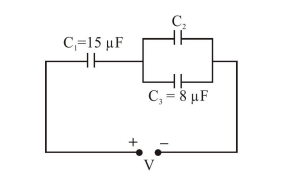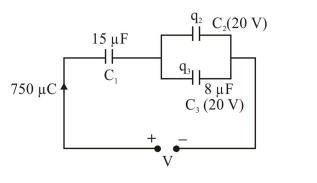# In the circuit shown in the figure,

Question:

In the circuit shown in the figure, the total charge in $750 \mu \mathrm{C}$ and the voltage across capacitor $\mathrm{C}_{2}$ is $20 \mathrm{~V}$. Then the charge on capacitor $\mathrm{C}_{2}$ is :1. $590 \mu \mathrm{C}$

2. $450 \mu \mathrm{C}$

3. $650 \mu \mathrm{C}$

4. $160 \mu \mathrm{C}$

Correct Option: 1

Solution:$\mathrm{q}_{3}=20 \times 8=160 \mu \mathrm{C}$

$\therefore \mathrm{q}_{2}=750-160=590 \mu \mathrm{C}$